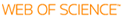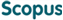Cited time inCited time inOn the Efficient Estimation of Min-Entropy

Title
On the Efficient Estimation of Min-Entropy
Author(s)
Kim, YongjuneGuyot, CyrilKim, Young-Sik
DGIST Authors
Kim, YongjuneGuyot, CyrilKim, Young-Sik
Issued Date
2021-04
Type
Article
Author Keywords
EntropyNISTEstimationComputational complexityClosed-form solutionsCryptographyRandom variablesEntropy estimationmin-entropyShannon entropyR&#233nyi entropyNIST SP 800-90Bcompression estimatorrandom number generator
ISSN
1556-6013
Abstract
The min-entropy is a widely used metric to quantify the randomness of generated random numbers in cryptographic applications; it measures the difficulty of guessing the most likely output. An important min-entropy estimator is the compression estimator of NIST Special Publication (SP) 800-90B, which relies on Maurer’s universal test. In this paper, we propose two kinds of min-entropy estimators to improve computational complexity and estimation accuracy by leveraging two variations of Maurer’s test: Coron’s test (for Shannon entropy) and Kim’s test (for Rényi entropy). First, we propose a min-entropy estimator based on Coron’s test. It is computationally more efficient than the compression estimator while maintaining the estimation accuracy. The secondly proposed estimator relies on Kim’s test that computes the Rényi entropy. This estimator improves estimation accuracy as well as computational complexity. We analytically characterize the bias-variance tradeoff, which depends on the order of Rényi entropy. By taking into account this tradeoff, we observe that the order of two is a proper assignment and focus on the min-entropy estimation based on the collision entropy (i.e., Rényi entropy of order two). The min-entropy estimation from the collision entropy can be described by a closed-form solution, whereas both the compression estimator and the proposed estimator based on Coron’s test do not have closed-form solutions. By leveraging the closed-form solution, we also propose a lightweight estimator that processes data samples in an online manner. Numerical evaluations demonstrate that the first proposed estimator achieves the same accuracy as the compression estimator with much less computation. The proposed estimator based on the collision entropy can even improve the accuracy and reduce the computational complexity. IEEE
URI
http://hdl.handle.net/20.500.11750/13820
DOI
10.1109/tifs.2021.3070424
Publisher
Institute of Electrical and Electronics Engineers
Files in This Item:

There are no files associated with this item.

Appears in Collections:
Department of Electrical Engineering and Computer Science Information, Computing, and Intelligence Laboratory 1. Journal Articles